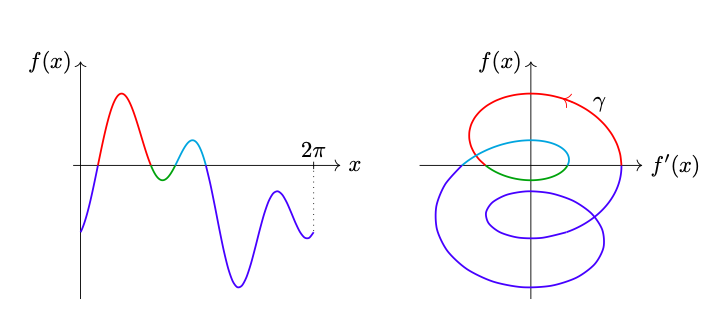# Counting the Solutions of an Equation without Solving itA basic task in mathematics or mathematical modelling consists in solving equations. Since it is often times impossible to solve equations analytically, one is interested in understanding properties of the solution space of the equation, e.g. its cardinality. In this work we construct a method that allows to count the number of solutions of a real equation in one real variable without solving the equation. Based on geometric ideas, we construct an integral that counts the number of solutions to an equation under mild regularity assumptions.

N. Hungerbühler, M. Wasem, An integral that counts the zeros of a function, Open Math, 2018